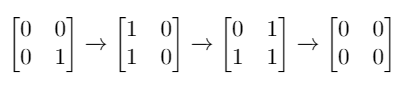# 转化为全零矩阵的最少反转次数

## 1284. 转化为全零矩阵的最少反转次数 (Hard)```输入：mat = [[0,0],[0,1]]

```

```输入：mat = []

```

```输入：mat = [[1,1,1],[1,0,1],[0,0,0]]

```

```输入：mat = [[1,0,0],[1,0,0]]

```

• `m == mat.length`
• `n == mat.length`
• `1 <= m <= 3`
• `1 <= n <= 3`
• `mat[i][j]` 是 0 或 1 。

### 相关话题

[位运算] [广度优先搜索] [数组] [矩阵]